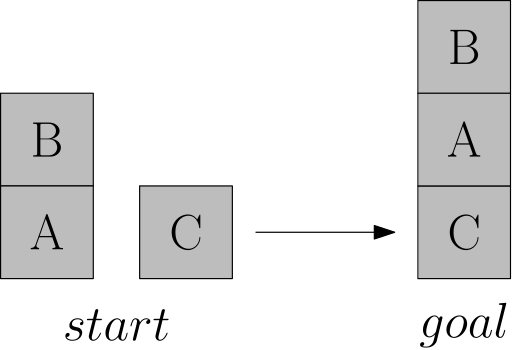# Task 1: BlockWorld, A* and Heuristics

## Henwas' trouble

For many years, in an old dock, there worked a man named Henwas, whose job was to move crates. Every shippment arrived in a scrambled order and according to the old man's notes, he was to rearrange it into a new configuration. As the man aged, his back started to hurt and he was unwilling to do any superfluous work. Hence, he reached to you to help him ease his pain. Help poor Henwas to find an optimal solution for his problem!

Recently, a shippment of three crates arrived. On their side there are labels A, B and C. Henwas looked into his notes and found that the final configuration should be B-A-C:To save space, the notes also use a succinct code to describe the configurations. This is the same as the image above:

[[B, A], [C]] -> [[B, A, C]]

Henwas felt quite confident, so he did not wait for you and immediately started moving the crates. Obviously, he could move only the crates which are on top of any stack. He can also use all the ground space around. To remember what he did, he uses 0 to denote the ground and write the actions as follows:

[[B, A], [C]] -> [[B, A, C]]
--------------------------
(B, 0) -> [[B], [A], [C]]
(A, C) -> [[B], [A, C]]
(B, A) -> [[B, A, C]]

Note that the order of stacks does not matter:

[[B, A], [C]] == [[C], [B, A]]

Important: Proběhla mírná změna v zipu níže - nové referenční problémy (váš kód zůstává nezměněn). Prosím o stažení nové verze 2 (9. 3. 2021, 12:00).

And that's where you come in! Given an start and goal configuration, your job is to write an algorithm that finds an optimal sequence of actions to reconfigure start to goal. Fortunatelly, most of the work is done and you can download it here: task1_blockworld_v2.zip. The only thing you have to do is implement the A* algorithm and the problem's heuristic.

You can play with the environment by directly running python blockworld.py as follows:

[[1, 2], ] -> [[3, 2, 1]]

$python blockworld.py state = [[1, 2], ] actions = [(1, 0), (1, 3), (3, 1)] <from> <to>: 1 0 state = [, , ] actions = [(2, 3), (2, 1), (3, 2), (3, 1), (1, 2), (1, 3)] <from> <to>: 2 1 state = [, [2, 1]] actions = [(3, 2), (2, 0), (2, 3)] <from> <to>: 3 1 !invalid action cannot move to where state = [, [2, 1]] actions = [(3, 2), (2, 0), (2, 3)] <from> <to>: 3 2 state = [[3, 2, 1]] Now, when you open student.py, you'll see the following: from blockworld import BlockWorld class BlockWorldHeuristic(BlockWorld): def __init__(self, num_blocks): BlockWorld.__init__(self, num_blocks) def heuristic(self, goal): self_state = self.get_state() goal_state = goal.get_state() # ToDo. Implement the heuristic here. return 0. class AStar(): def search(self, start, goal): # ToDo. Return a list of optimal actions that takes start to goal. # You can access all actions and neighbors like this: # for action, neighbor in state.get_neigbors(): # ... return None Implement the heuristic in BlockWorldHeuristic::heuristic() and AStar in AStar::search(). After you are done, you can test your algorithm with prepared instances, available in problems/<N>/<PID> folders. You can evaluate your algorithm with python eval.py <N> <PID>: $ python eval.py 7 0
7/0: [, [6, 3], , [5, 1, 4]] -> [[7, 3, 5, 2, 1, 6], ]
Reference | path length: 7, expanded: 1417, time: 1.26s
Yours     | path length: 7, expanded: 1417, time: 1.14s

Hint: You can use Python's PriorityQueue as follows:

from queue import PriorityQueue

queue = PriorityQueue()

queue.put( (3, "Nimue") )
queue.put( (1, "Crystin") )
queue.put( (2, "Rhys") )

while not queue.empty():
priority, name = queue.get()
print(f"{priority} - {name}")

Which prints

1 - Crystin
2 - Rhys
3 - Nimue

## Evaluation

Upload your solution (only student.py) into Brute, where it will be evaluated automatically on a set of problems with N = 5, 6, 7, 8, 9. For each N, you will get 1 points if you provide valid solutions, i.e., the actions take the start state to the goal. Further, you'll gain 1 point if the solutions are optimal, for each N. Each solved problem has a time limit.

(note for next year: get_neigbors → get_neighbors; update student package)